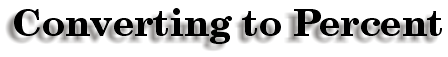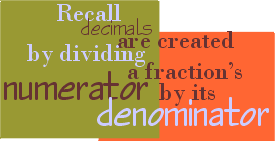Example: Consider the fraction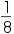1÷8 = .125 We now have 1/8 represented as the decimal .125. Now we change .125 into 12.5% by multiplying by 100 or moving the decimal place 2 places to the right.

At Gem High School, 7 out of every 9 students voted for Mr. Diamondstone as the best teacher in the school.
What percent voted for Mr. D?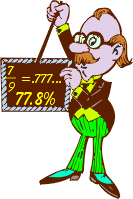First we write 7 out of 9 as the fraction 7 / 9.

To get a decimal representation, we divide 7 by 9 and get .777…

We multiply by 100 or move the decimal place two places to the right to get 77.8% (note that we rounded to the nearest tenth of a percent).
It is important to note that:

7 / 9 = .777…= 77.8%

 These representations are equal, but it depends on the circumstances to decide when one would use each representation.

 Of 9 students surveyed, we wanted to know how many liked Mr. Diamondstone. On average, we could say that 7 liked him, and would vote for Mr. D.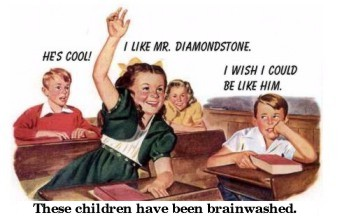However, if we were talking baseball, and Junior got 7 hits out of 9 at bats, we would use the decimal .778 to represent his average. If we were in school and J.J. got 7 questions correct out of 9, we would use the percent 77.8 to represent his grade.Converting from Percent to Fractions

 writer:Kevin Gallagher

Percent